# PyCaret 2.3.5 Is Here! Learn What’s New

By Moez Ali, Founder & Author of PyCaret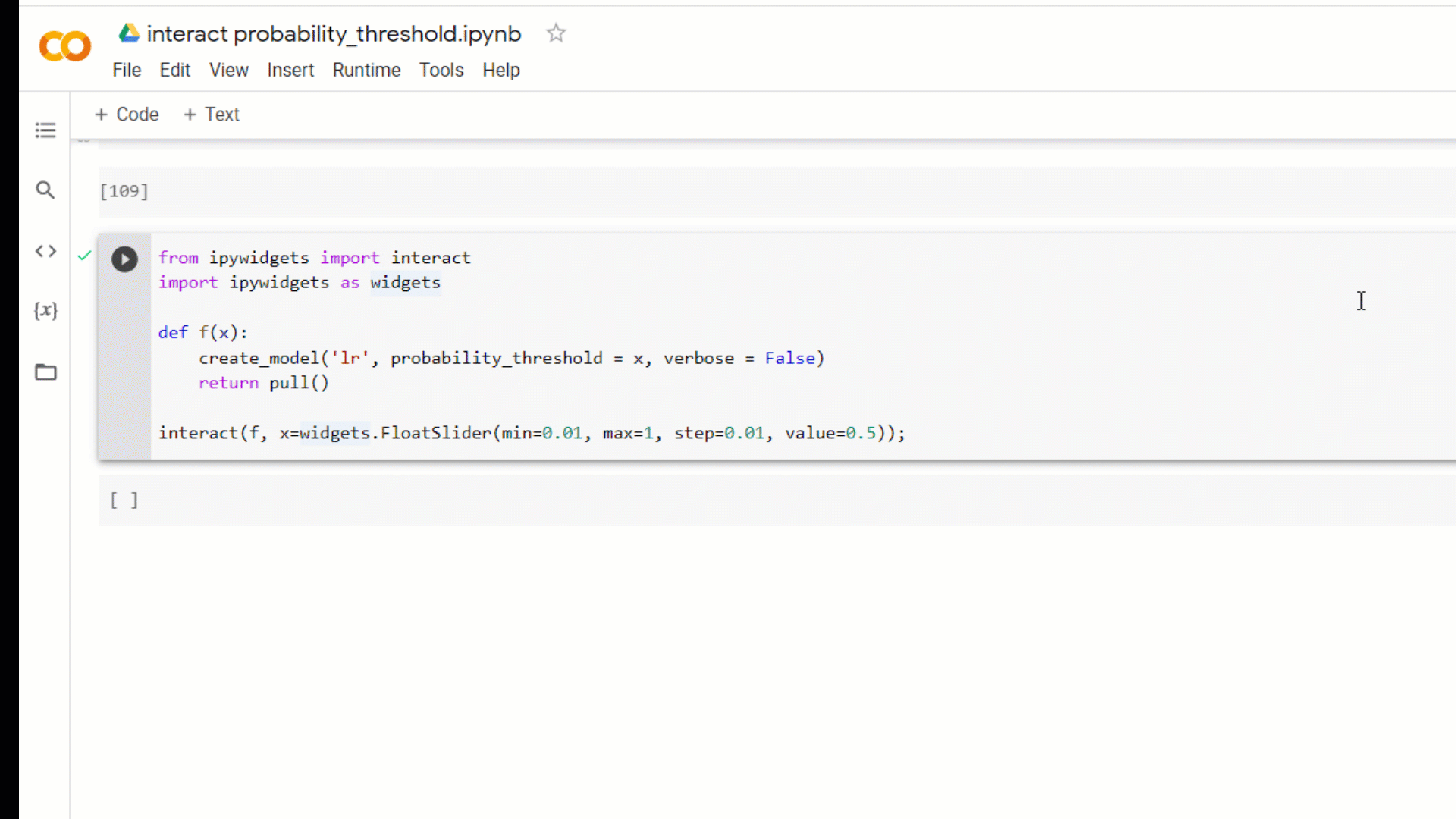(Image by Author) A new feature in PyCaret 2.3.5

## 🚀 Introduction

PyCaret is an open-source, low-code machine learning library in Python that automates machine learning workflows. It is an end-to-end machine learning and model management tool that speeds up the experiment cycle exponentially and makes you more productive. To learn more about PyCaret, you can check the official website or GitHub.

## 🤖 New Models: DummyClassifier and DummyRegressor

DummyClassifier and DummyRegressor are added in the model zoo of `pycaret.classification` and `pycaret.regression` modules. When you run `compare_models` it will train a dummy model (classifier or regressor)using simple rules and the results will be shown on the leaderboard for comparison purposes.

``````# load dataset
from pycaret.datasets import get_data
data = get_data('juice')# init setup
from pycaret.classification import *
s = setup(data, target = 'Purchase', session_id = 123)# model training & selection
best = compare_models()``````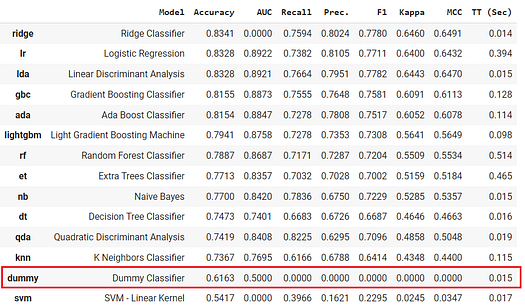(Image by Author) — Output from the compare_models function

``````# load dataset
from pycaret.datasets import get_data
data = get_data('boston')# init setup
from pycaret.regression import *
s = setup(data, target = 'medv', session_id = 123)# model training & selection
best = compare_models()``````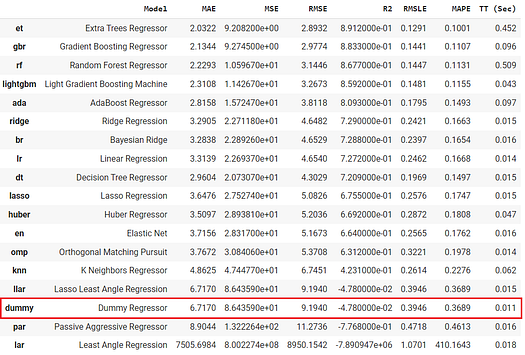(Image by Author) — Output from the compare_models function

You can also use this model in the `create_model` function as well.

``````# train dummy regressor
dummy = create_model('dummy', strategy = 'quantile', quantile = 0.5)``````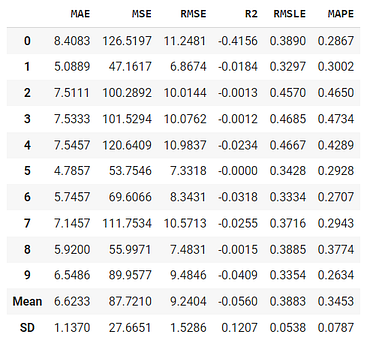(Image by Author) — Output from the create_model function

Dummy models (classifier or regressor) are useful as a simple baseline to compare with other (real) regressors. Do not use it for real problems.

## 📈 Custom Probability Cut-off

A new parameter `probability_threshold` is introduced in all the training functions of PyCaret such as `create_model` `compare_models` `ensemble_model` `blend_models` , etc. By default, all the classifiers that are capable of predicting probabilities use 0.5 as a cut-off threshold.

This new parameter will allow users to pass a float between 0 and 1 to set a custom probability threshold. When `probability_threshold` is used, the object returned by the underlying function is a wrapper of the model object, which means that when you pass it in the `predict_model` function, it will respect the threshold and will use them to generate hard labels on the passed data.

``````# load dataset
from pycaret.datasets import get_data
data = get_data('juice')# init setup
from pycaret.classification import *
s = setup(data, target = 'Purchase', session_id = 123)# model training
lr = create_model('lr')
lr_30 = create_model('lr', probability_threshold = 0.3)``````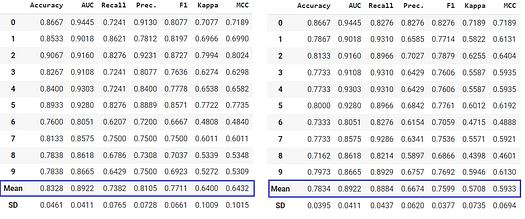(Image by Author) Left side- LR with no probability threshold, uses default 0.5 | Right Side — LR with probability_threshold = 0.3.

You can write a simple loop like this to optimize probability cut-offs:

``````# train 10 models at diff thresholdsrecalls = []for i in np.arange(0,1,0.1):
model = create_model('lr', probability_threshold = i, verbose=False)
recalls.append(pull()['Recall']['Mean'])# plot it
import pandas as pd
df = pd.DataFrame()
df['threshold'], df['recall'] = np.arange(0,1,0.1), recalls
df.set_index('threshold').plot()``````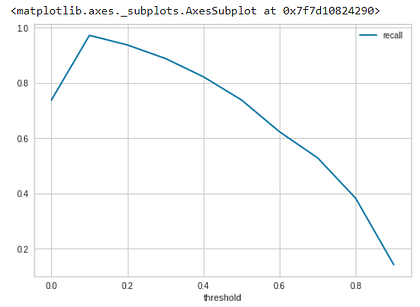(Image by Author) Recall at different probability_threshold (x-axis is the threshold, the y-axis is the recall)

You can also simply build an `ipywidgets`dashboard to test out different probability thresholds for different models.

``````from ipywidgets import interact
import ipywidgets as widgetsdef f(x):
create_model('lr', probability_threshold = x, verbose = False)
return pull()interact(f, x=widgets.FloatSlider(min = 0.01, max = 1.0, step = 0.01, value = 0.5));``````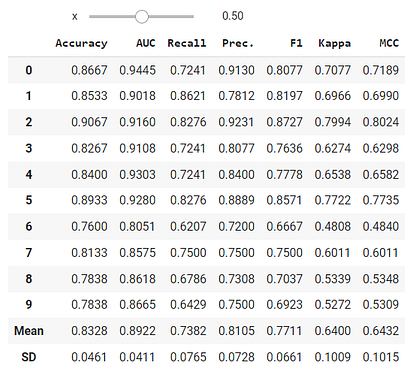(Image by Author) — As you change the value of the slider, the model will be re-trained, and the CV results will be updated in real-time.

Notebook with all the code examples shown in this announcement is in this Google Colab Notebook.

⭐ Tutorials New to PyCaret? Check out our official notebooks!
📋 Example Notebooks created by the community.
📙 Blog Tutorials and articles by contributors.
📚 Documentation The detailed API docs of PyCaret
📺 Video Tutorials Our video tutorial from various events.
📢 Discussions Have questions? Engage with community and contributors.
🛠️ Changelog Changes and version history.
🌳 Roadmap PyCaret’s software and community development plan.

Bio: Moez Ali writes about PyCaret and its use-cases in the real world, If you would like to be notified automatically, you can follow Moez on Medium, LinkedIn, and Twitter.

Original. Reposted with permission.

Related: